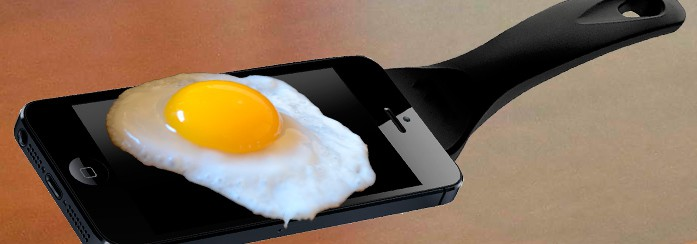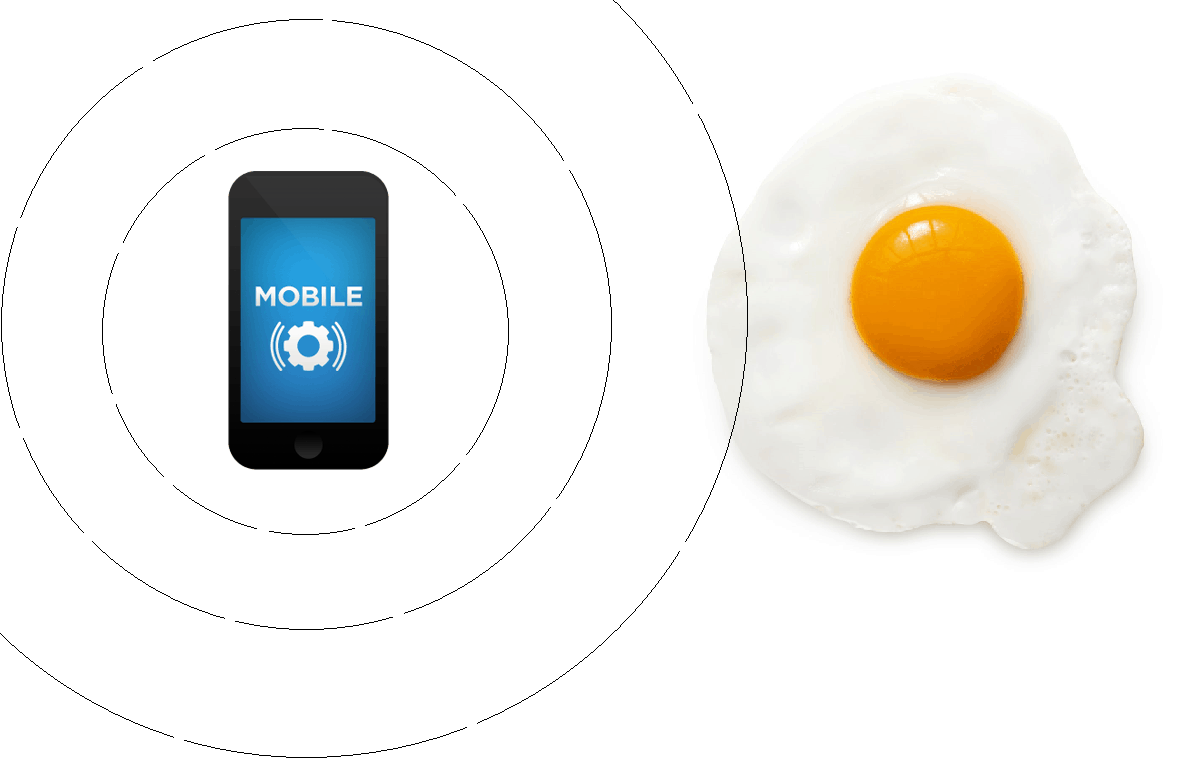# Can my Mobile Cook an Egg? Hardly ever!Although all representatives of modern technologies have more advantages than negative sides, you have to mind the implicit harm they emit. Just imagine how much it matters if two smarties can  cook an egg (according to this article). Wanna your brain scramble?.. Yup, take your mom away from the screen as she’ll definitely take away your phone. But wait, is it reality or just a trick? Here we’re going to destroy this myth made up by amateurs. Our team of experts has conducted a research for those guys who enjoy daily talking on the phone. Simple calculations we’re going to show will convince you of the fact that your gadget is not that harmful.

To to start with, we assume emitting power of a mobile phone is $300 \, mW$ (as stated in this article). This video (fake, as you’ll see later) “demonstrates” cooking an egg with $9$ cell phones:

Let’s analyze this situation. For $9$ mobiles emitting power $P$ comes as follows:

$P=300\,mW \times 9=2700\, mW=2.7\, W$

We round this up to $3$ W to simplify calculations. To find out what’s the effect caused by mobiles on the egg, we apply the following formula:

$\Delta E=Q=m\times C \times \Delta T$

where $\Delta E=Q$ is energy consumption (amount of heat received by the egg), $m$ is mass of an egg, $C$ – heat capacity of an egg, $\Delta T$ – temperature change. Heat capacity of an average egg equals $3$ joule/(g*K). Thus, to provide $1$ degree temperature increase of $60 \, g$ egg, energy consumption should be:

$Q=3\times 60 \times 1=180 \, joules$

Now, we need to calculate in what time period our mobiles can provide such energy. As we know, energy is related with power due to the following formula:

$\Delta E=P\times t$

where $t$ is time. Thus, compound emissive power $P=3\, W$ of our mobiles can provide required energy amount within:

$t=\frac{Q}{P}=\frac{180}{3}=60\, sec$

The above result occurs on account that the whole mobile emission is absorbed. However, first of all, mobile phone spreads emission around and above, so only nearly $20$% come to an egg (in regard to angular size of a fried egg).It means that in fact egg catches:

$P\times 0.2=3 \, W\times 0.2=600 \, mW$

Secondly, emitting power of mobile phones meets standards of Specific Absorption Rate (SAR) in power absorbed by unit of mass. It stands for $2$ W in Europe. Hence, our egg absorbs less than:

$2\,W\times 60\, g=2\,W\times 0.06\, kg=0.12 \, W=120\, mW$

So, this is the real power $P_r$ absorbed by the egg. Hence, we need to reapply our formula for time. $P_r=120 \,mW$ heats the egg to $1$ degree per:

$t_r=\frac{Q}{P_r}=\frac{180}{0.12}=1500\, sec= 25\, min$

Obviously, we can’t fry the egg this way, especially when we take into account heat exchange with air and a frying pan. Don’t believe? Try it yourself!

Consequently, the answer is NO, your mobile actually can’t fry  the egg for you to have breakfast while talking, so let alone your brain, that’s good! (Probably, it can become possible if next year iPhone will have an application of this frying pan. Apple would become a real Eggl then). Yet, do not overuse mobiles anyway, as their overuse may harm some other body functions.

7 Shares
Filed under Engineering, Physics.